# Average scalar curvature

This article defines a notion of curvature for a differential manifold equipped with a Riemannian metric

This article defines a scalar value (viz, a real number) associated with a Riemannian manifold. This real number depends both on the underlying differential manifold and the Riemannian metric

## Definition

### Given data

A compact connected differential manifold$M$ with a Riemannian metric$g$.

### Definition part

The average scalar curvature of$M$ is defined as the volume-averaged value of the scalar curvature over the manifold. That is, if$R$ denotes the scalar curvature and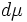$d\mu$ the volume element, we have that: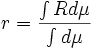$r = \frac{\int R d\mu}{\int d\mu}$

Here, the volume element$d\mu$ is the natural choice of volume-element arising from the Riemannian metric.

## Facts

### For surfaces

For the two-dimensional case, viz surfaces, in the special event that the surface is orientable, we can use the Gauss-Bonnet theorem to determine the average value of scalar curvature over the manifold. That is, we use the fact that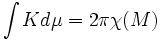$\int K d\mu = 2\pi\chi(M)$ where$\chi$ denotes the Euler characteristic, which is a topological invariant (and hence, specifically, a property intrinsic to the differential manifold).

Since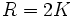$R = 2K$, this gives: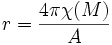$r = \frac{4\pi\chi(M)}{A}$

where$A$ denotes the total volume of the manifold.